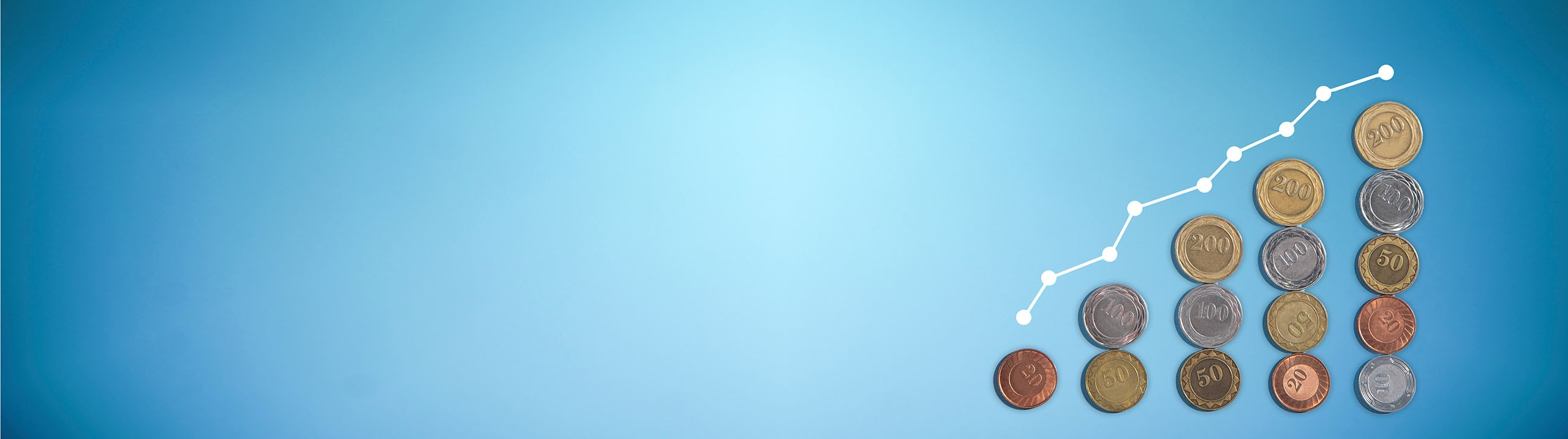## Macroeconomics

Learn the toughest concepts covered in your Macroeconomics class with step-by-step video tutorials and practice problems.

Monetary Policy

# Quantity Theory of Money

1
concept

## Quantity Theory of Money4m
Play a video:
Alright. So now let's look into the quantity theory of money. So the quantity theory of money, what it does is it connects the money supply? So the amount of money available in the economy with the level of prices? So there's gotta be some connection with how much money is available and how the price level is going to be in the economy. And that's what this theory is trying to connect. So it's a very simple equation. We've got M. Times V. Equals P times why? So let's go ahead and define what all of these are. M. Is our money supply. And remember we're trying to connect the money supply to the level of prices in the economy. So let's see what these variables do. So our money supply. The amount of money available in the economy set by the fed and then the velocity of money velocity of money. It's kind of a funny term. But what does that mean? It's the average number of times each dollar is spent in the money supply. So think about when you go to the store and you spend a dollar, you you bring a dollar and you buy a candy bar and then the store goes and they take that money and they pay their employees and the employee goes and buy some groceries at the store and then that dollar goes from the store and gets spent somewhere else, right? So the same dollar doesn't just get spent once and get retired. No it keeps circulating, it goes from one hand to another and keeps getting spent keeps getting spent, right? So the velocity of money is the average number of times each dollar is going to be spent. Okay. Generally in a year, how many times will this dollar that I have in my pocket? How many times will I spend it? Someone else spend it? How many times will it change hands during one year? That's the velocity of money. Next we have P which is the price level in the economy. And remember we're trying to connect the money supply to the level of prices and finally, why is going to be our GDP? Okay GDP in the economy? And we'll say Real GDP because remember Real GDP keeps prices constant and then we'll multiply it by the price level to get to the current prices in the economy. Alright, so let's go through this example together real quick and we'll see how this money supply works and then we'll go into more detail in the next video. So in 2014 the money supply equaled \$2.8 trillion, while real GDP totaled 16 trillion price deflator was 1.09, calculate the velocity of money. So remember we've got m times v The money supply times the velocity equals the price level, times the level of real GDP. Okay, so let's go ahead and put in what we know they told us the money supply is 2.8 trillion we'll just leave it as 2.8 And we're calculating the velocity of money, they told us the price deflator, price, the price level is 1.09. What does that 1.09 mean that means that prices are one point oh nine what they were in the original year? In our base year. So if we were thinking something cost us a dollar in the base year, well this year, that same product would cost us a dollar and nine cents. Okay, so the prices have gone up since the since the base year And real GDP, it also told us is 16 trillion. Okay, so we've got all of our variables except the velocity. Let's go ahead and solve for the velocity here. If we divide both sides by 2.8, Well we'll have solved for the velocity. So let's go ahead and snag our calculators. Let's do some quick math here. So 1.09 times 16 Divided by 2.8. So that tells us the velocity. I'm gonna I'm gonna round it off to six here. I'm just gonna say it's approximately six, it was like six point something and it comes out to approximately six. So that says each dollar changes hands approximately six times per year, Okay, per year. So each time you spend a dollar it's going to be spent on average six times each dollar in the economy. Right? So that's how the theory works. You'll be given some variables and you can solve for the last variable. Um But let's go ahead and think a little bit more of the implications of the quantity theory.
2
concept

## Quantity Theory of Money and Inflation5m
Play a video: